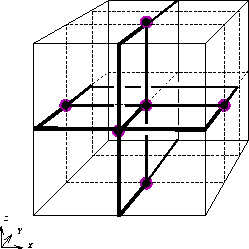# Computational Geometry Research at McGill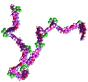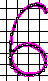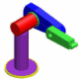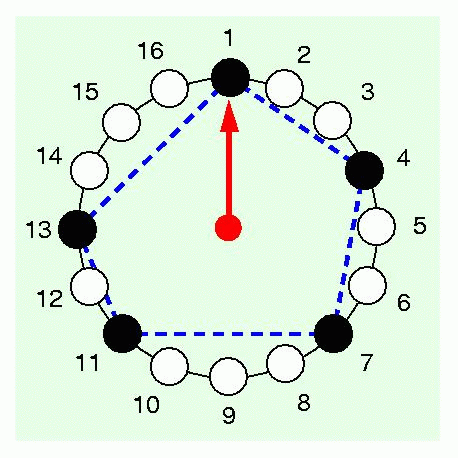Molecules Fonts Motion Planning Music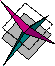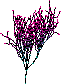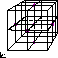Manufacturing Probability Polyhedral Computation Graph Drawing## Molecular Reconfiguration

In chemistry and physics large molecules are often modelled as polygonal linkages. Properties of these molecules can be determined by geometric properties of the underlying linkages. The purpose of this research is to study the combinatorial and algorithmic aspects of polygonal linkages to help further our understanding of how molecules reconfigure themselves.

Godfried Toussaint

## Font Design

In font design, we study the geometric, mathematical and methodological problems associated with the simulation of handwriting, random typefaces, brushed characters, running ink and linked letters. The character "6" on this page is from a typeface designed from a sample written on a magnetic pad. Our software identifies the important points of a stroke, creates smooth Bézier curves, defines glyphs based on various pen nibs, and outputs a Postscript font.

Luc Devroye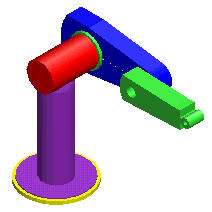## Robot Motion Planning

Robotic arms are frequently used in manufacturing plants to perform tasks such as welding, screwing and drilling. Geometric questions in robotic motion planning involve finding efficient motion plans for a given robotic arm and determining what kinds of tasks a given robotic arm can and can not do.

Sue Whitesides

## Computational Geometric Problems in Music

Symbolic music, particularly rhythm and melody, are studied from the geometric and algorithmic points of view. In particular, we are interested in
(1) methods for visualizing, representing, and comparing the similarity of rhythms and melodies,
(2) developing mathematical measures of syncopation,
(3) applying music theory to ethnomusicology,
(4) applying pattern recognition tools to music information retrieval, and
(5) applying tools from bioinformatics, such as phylogenetic analysis, to aid in the determination of a phylogeny of world rhythms.## Geometric Problems in Manufacturing

There are many interesting geometric problems that arise in manufacturing. A typical example of the problems we study is the problem of finding a stable grip on an object with a robotic hand consisting of two parallel rectangular plates. The diagram on the left illustrates a particularly challenging case.

Godfried Toussaint

## Probabilistic Analysis

We study the expected behavior of algorithms and data structures under random input or artificial randomization. Our work builds on elementary probability theory rather than combinatorial analysis. Subtopics of particular interest include random number generation and random trees. For example, we create models of random trees for simulating virtual forests. The tree on this page is a binary search tree built from a Weyl sequence (e), (2e), (3e), etcetera, where (.) denotes modulo 1.

Luc Devroye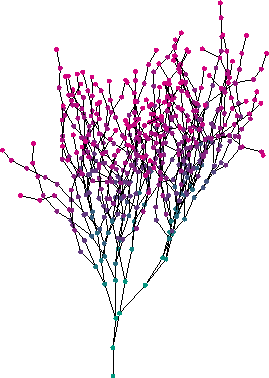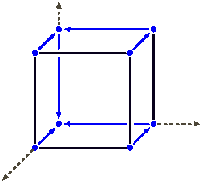## Polyhedral Computation

The theory of convex polytopes has its origins in the study of systems of linear inequalities. A (convex) polyhedron is the set of solutions to a system of linear inequalities. A bounded polyhedron is called a polytope. The vertices of a polytope are those feasible points that do not lie in the interior of a line segment between two other feasible points. Converting from the halfspaces to the vertices is called vertex enumeration. An important open problem is the existence of a vertex enumeration algorithm polynomial in the number of halfspaces plus the number of vertices. We distribute the lrs library which performs a number of related functions, including vertex/facet enumeration, redundancy removal, computing Nash equilibria and Fourier elimination. We are also interested in applications of polyhedral computation to a wide number of areas, most recently to quantum information.

David Avis

## Graph Drawing

We study the layout of graphs and diagrams. For example, the picture here illustrates a 3-dimensional orthogonal grid layout of a graph.

Sue Whitesides